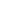#Financial Math Basics is a Course

## Financial Math Basics

Time limit: 90 days

### Full course description

Mathematics is a foundational pillar of business and finance. It is necessary to understand how to apply math in different financial situations to have business success. This course will allow you to strengthen your math skills by providing examples of how basic math concepts apply in a variety of financial settings, including calculating interest, business expenses, and employee compensation.

Learning Outcomes:

Apply concepts of percentages to calculate percent change, discounted prices, and sales tax

Distinguish between markup percentages and profit margins

Calculate interest including simple interest and compound interest

Compute the annual percentage yield (APY) and annual percentage rate (APR) in different situations

Compute the present value and future value of an investment

Calculate interest for installment buying and open-end credit

Compute annual depreciation using different methods of depreciation

Understand how net wages are calculated for employees

Evaluate stock investments using different financial ratios

Credits:

IACET CEUs: 0.6 (Contact Hours: 6 hours)

Estimated time to complete: 6 hours